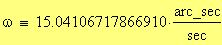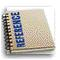EARTH SCIENCE
Earth StatisticsThe Earth can be described mathematically as a spherical object with the following parameters: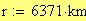Equatorial circumference: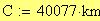Volume: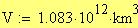Mass: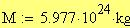Average density: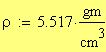The Earth's interior is divided into three parts: the crust, the mantle, and the core. The Earth's crust is 6 km deep under the oceans but about 30 km deep under land. Its mantle extends from 30 km to 2900 km; and its core extends from 2900 km to 6378 km, which is the center of the Earth.
Maximum depth of crust: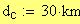Maximum depth of mantle: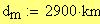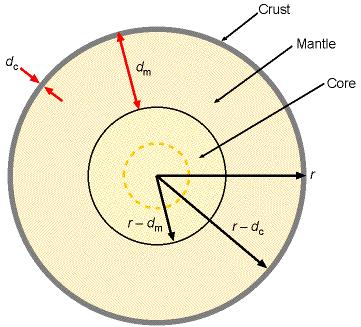The interior temperature of the Earth rises steadily from the Earth's surface to its center. The temperature reaches 6000oC near the center of the Earth.
The Earth is not a perfect sphere; it is flattened out at its poles as a result of rotation. Hence, the radius of the Earth varies with position.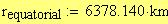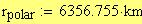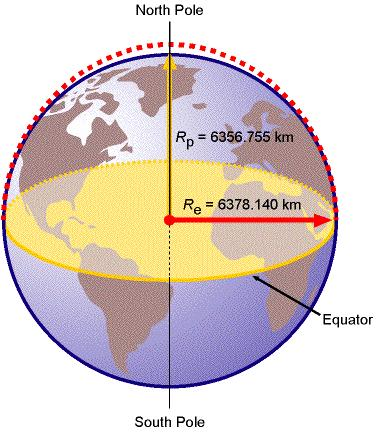The Earth rotates around the Sun in an elliptical orbit.
Average Earth-Sun distance: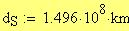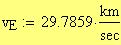Average orbital speed:
Average centripetal acceleration: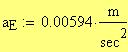Rotational period:
in mean sidereal time: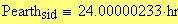in mean solar time: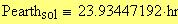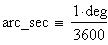Rate of rotation: